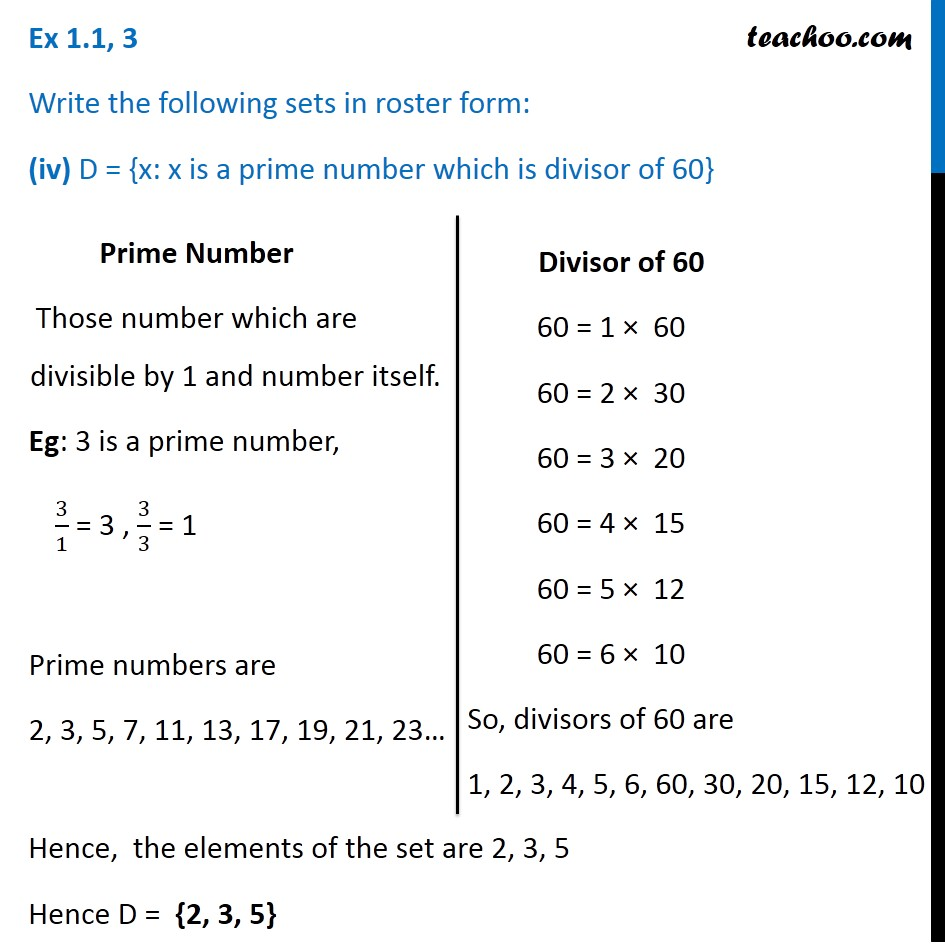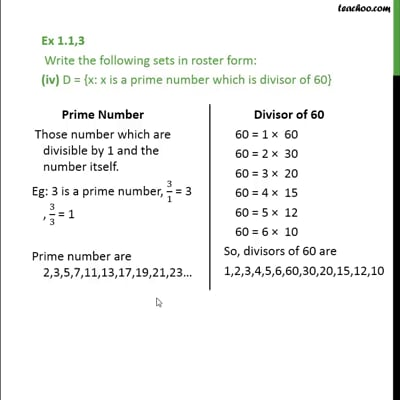Ex 1.1

Chapter 1 Class 11 Sets
Serial order wiseThis video is only available for Teachoo black users

Maths Crash Course - Live lectures + all videos + Real time Doubt solving!

### Transcript

Ex 1.1, 3 Write the following sets in roster form: (iv) D = {x: x is a prime number which is divisor of 60} Hence, the elements of the set are 2, 3, 5 Hence D = {2, 3, 5} Prime Number Those number which are divisible by 1 and number itself. Eg: 3 is a prime number, 3/1 = 3 , 3/3 = 1 Prime numbers are 2, 3, 5, 7, 11, 13, 17, 19, 21, 23… Divisor of 60 60 = 1 × 60 60 = 2 × 30 60 = 3 × 20 60 = 4 × 15 60 = 5 × 12 60 = 6 × 10 So, divisors of 60 are 1, 2, 3, 4, 5, 6, 60, 30, 20, 15, 12, 10